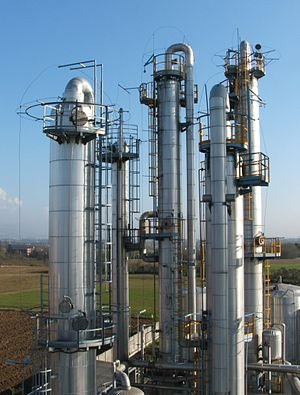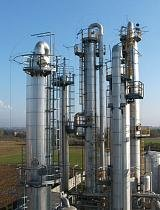Nature and Environment 5391 Views by Richard Nelson

### Introduction to Chemical Engineering

••One of the most important fields of engineering is chemical engineering. This field is part of the everyday lives of most citizens and will be for the foreseeable future. The amount of chemistry and math that is used in the field is staggering. The most important equation can be considered the mass balance.

(1)   Min = Mout

The mass balance has many complex forms but this is the simplest way of understanding nearly any chemical engineering process and that is that the mass that comes into a reactor in usually equal to the mass coming out of a reactor. This can be hard to recognize under normal conditions for instance with the common chemical reaction or hydrogen gas and oxygen gas to produce water.

(2)   2H2 + O2→2H2O

The problem that occurs is with changes for gases to liquids. This will not allow for the proper mass balance if it is not accounted for. For instance if the chemical engineer was to consider taking the masses of both sides of fluids they would quickly run into the problem that H2 and O2 naturally occur as gases and would not be able to balance the mass balance equation. Plus many forms of engineering consider the use of pipes and must take into account the equation for a circle to balance the mass equations.

(3) A=(pi)r^2

where

A = Area of the circle

r = radius of the circle

The most important equation that any math user must learn and chemical engineers are no exception is called the Pythagoras equation.

(4)   a2 + b2 = c2

This equation can be rearranged to follow nearly any form of math but in this form the “a” refers to the bottom of a right triangle, the b is the side, and c is the length of the hypotenuse or the diagonal side of the right triangle. This can come in handy in many situations for engineers but really helps with the physical explanations of many fundamentals learned in chemical engineering.